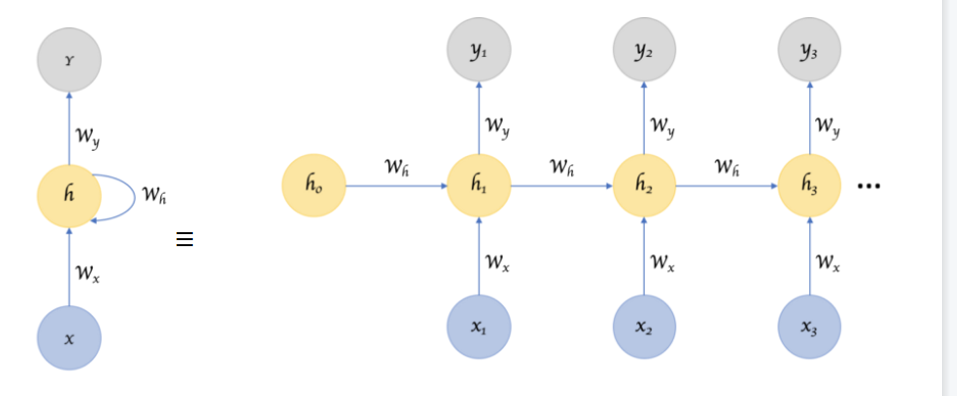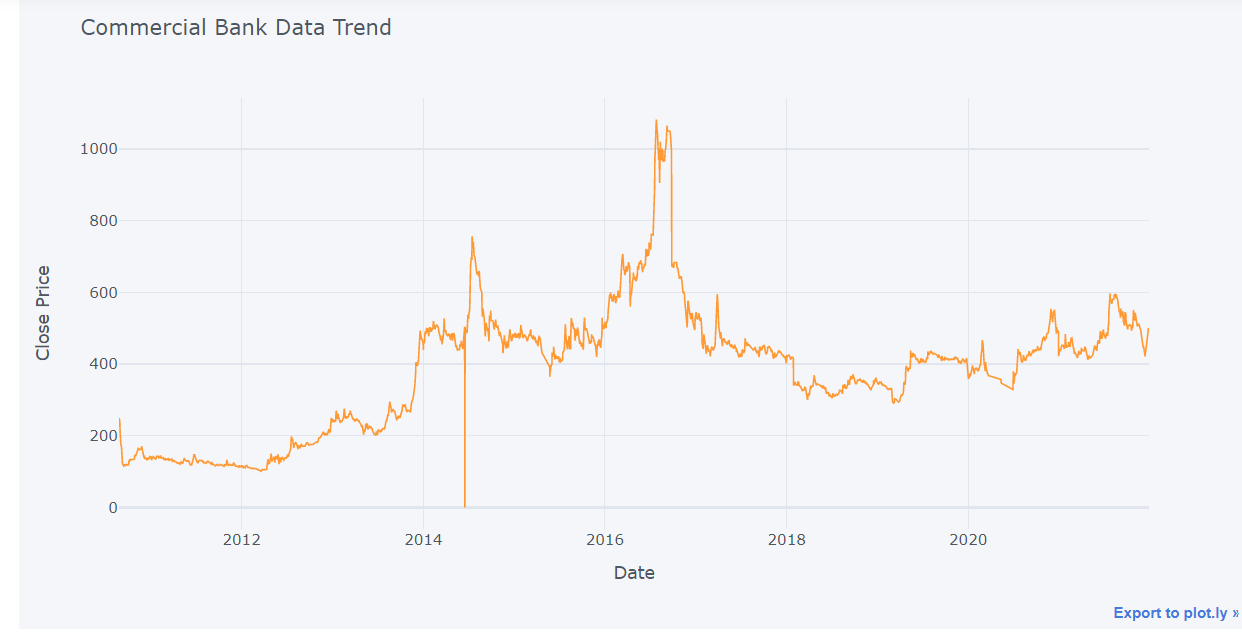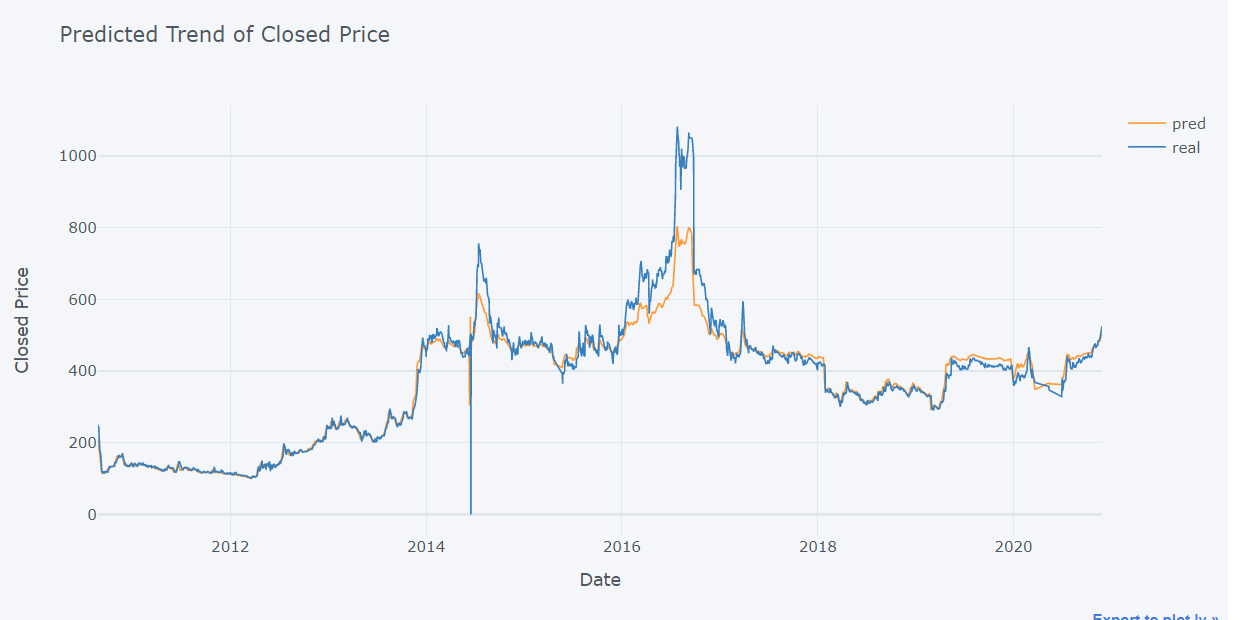# Basic Introduction to RNN

A type of neural network called a recurrent neural network (RNN) uses the output from a previous step as input for the current step. Traditional neural networks have inputs and outputs that are independent of one another, but there is a need to remember the previous words in situations where it is necessary to anticipate the next word in a sentence. As a result, RNN was developed, which utilized a Hidden Layer to resolve this problem. The Hidden state, which retains some information about a sequence, is the primary and most significant characteristic of RNNs. Following figure show the architecture of RNN.# Import

Here we import necessary module which we required while Fitting LSTM model on Commercial data.

pandas : For data analysis and data manipulation

numpy : for array manipulation and able to work with array type data

matplotlib: For data visualization and analysis of data

cufflinks : for making interactive plot with pandas data

sklearn : to do necessary preprocessing

keras : for RNN model build

import numpy as np
import pandas as pd
from sklearn.metrics import accuracy_score
from sklearn.preprocessing import StandardScaler
from keras.models import Sequential
from keras.layers import Dense, SimpleRNN



This specific data is acquired from GitHub, which houses numerous csv files from various businesses. However, I used data from a commercial bank for this LSTM model. There are 2572 rows in all.

commerce = pd.read_csv("ADBL_2000-01-01_2021-12-31.csv", engine='python')


S.N. Date Total Transactions Total Traded Shares Total Traded Amount Max. Price Min. Price Close Price
0 1 2021-12-29 1013 200533.0 98526860.8 499.0 488.0 492.0
1 2 2021-12-28 659 91046.0 44737396.5 498.0 488.0 494.0
2 3 2021-12-27 816 88858.0 44186856.3 510.0 491.0 493.0
3 4 2021-12-26 1002 130801.0 65306688.9 502.0 495.0 500.0
4 5 2021-12-23 714 77234.0 38044401.2 497.0 481.0 496.0
commerce.shape

(2572, 8)


# Sort Data in Ascending Order

Our data is arranged according to time in descending order. In order to maintain our data in rising order according to our date property, we here change the given data into ascending order. Using the built-in function head, we were able to only examine a portion of the given data set (). We can only access the top 5 data points of the provided dataset by utilizing this head function.

commerce = commerce.sort_values(by="Date")

S.N. Date Total Transactions Total Traded Shares Total Traded Amount Max. Price Min. Price Close Price
2571 2572 2010-08-26 2 40.0 10150.0 255.0 250.0 250.0
2570 2571 2010-08-29 2 20.0 4860.0 245.0 241.0 241.0
2569 2570 2010-08-30 6 60.0 13620.0 237.0 217.0 217.0
2568 2569 2010-08-31 6 60.0 12210.0 213.0 196.0 196.0
2567 2568 2010-09-02 6 60.0 11130.0 193.0 178.0 178.0

# Handle Missing NAs

Our analysis might not be successful if there are any missing values. Therefore, we need look for missing values to make our data clean and able to be evaluated. If necessary, we can substitute the missing value with the prior or following value using the average of the other values. However, we ignore the missing data in this instance.

commerce = commerce.dropna()
commerce.tail()

S.N. Date Total Transactions Total Traded Shares Total Traded Amount Max. Price Min. Price Close Price
4 5 2021-12-23 714 77234.0 38044401.2 497.0 481.0 496.0
3 4 2021-12-26 1002 130801.0 65306688.9 502.0 495.0 500.0
2 3 2021-12-27 816 88858.0 44186856.3 510.0 491.0 493.0
1 2 2021-12-28 659 91046.0 44737396.5 498.0 488.0 494.0
0 1 2021-12-29 1013 200533.0 98526860.8 499.0 488.0 492.0

# Plot Trend

Here, we plot our statistics to see how they are trending. by keeping the data in the line plot’s horizontal axis and the closing price in its vertical axis. We are aware that time series data is information that varies over time. As a result, they lack a steady trend.

commerce.iplot(kind="line",x="Date",y="Close Price",xTitle="Date", yTitle="Close Price", title="Commercial Bank Trend")The graph shows that the initial closure price was around 270, and that it subsequently declined before gradually rising to its ideal level in July 2020, which was around 1200. Throughout the remaining time frame, there are frequent changes in closing price.

# Next Price

To obtain the next price we shift close price one step back. Because tomorrow closed price is next price for today. And in next step we drop the missing values.

commerce['Next Price'] = commerce.shift(-1)['Close Price']

commerce = commerce.dropna()


# Rename Columns

We changed the column names of the provided dataset because some of the original data’s columns weren’t initialized in the typical order; this made various manipulation chores easier.

commerce.rename(columns = {'S.N.':'SN','Total Transactions':'TTrans', 'Total Traded Shares':'TTS',
'Max. Price':'MaxPrice','Min. Price':'MinPrice'}, inplace = True)


# Prepared Feature

Here we take Closeprice and Date as a features and NextPrice and Date as outfeature.

features = ['ClosePrice',"Date"]
outFeature = ['NextPrice',"Date"]


Here we assign variable X and Y to the features and outFeasture variable.

# take close price as feature and next price as out feature
X, Y = commerce[features], commerce[outFeature]
X.set_index("Date", inplace=True)
Y.set_index("Date", inplace=True)


# Normalize Data

Here, minmax normalization is used. To alter our data on the same scale, data standardization is increasingly necessary. Data normalization is therefore a crucial stage in the process of converting all data to the same measuring unit.

#Normalize data using standard scalar.
ss = StandardScaler()

X["ClosePrice"] = ss.fit_transform(X)
Y["NextPrice"] = ss.fit_transform(Y)



# Train Test Split

Data will be randomly split using train test split of sklearn, losing the sequence. As a result, we created scratch sets for our train and test datasets. We divided the data as follows: we used 80% of the data for the train set, 10% for the validation set, and the final 10% for the test set.

def train_test_split(x,y,train_size):
n = int(len(x)*train_size)
trainx = x[:n]
testx = x[n:]
trainy=y[:n]
testy=y[n:]
return trainx,testx,trainy,testy

#Split train, validation, test set from X,Y to 80%, 10%, 10%

X_train, X_test, Y_train, Y_test = train_test_split(X, Y, train_size=0.9)

X_train.shape,X_test.shape,X.shape

((2313, 1), (258, 1), (2571, 1))


# Convert to Matrix

Here, we convert train, test set of data into matrix inorder to do calculation easy.

# convert into dataset matrix
def convertToMatrix(data, step):
data = data.to_numpy()
X, Y =[], []
for i in range(len(data)-step):
d=i+step
X.append(data[i:d,])
Y.append(data[d,])
return np.array(X), np.array(Y)

step=5

trainX,trainY =convertToMatrix(X_train,step)
testX,testY =convertToMatrix(X_test,step)
# validX,validY = convertToMatrix(X_valid,step)

trainX = np.reshape(trainX, (trainX.shape, 1, trainX.shape))
# validX = np.reshape(validX, (validX.shape, 1, validX.shape))
testX = np.reshape(testX, (testX.shape, 1, testX.shape))

trainX.shape,testX.shape #,validX.shape

((2308, 1, 5), (253, 1, 5))


# Modeling

Here, we are going to build RNN model using simpleRNN which has 32 input unit and relu activation function in input layer similarly we used 8 hidden layer and relu as hidden activation function. We compile the model using rmsprop as optimizer function and mean_squared_error as a loss. Rest of this is we can see following.

# SimpleRNN model
model = Sequential()
model.compile(loss='mean_squared_error', optimizer='rmsprop')
model.summary()

Model: "sequential"
_________________________________________________________________
Layer (type)                Output Shape              Param #
=================================================================
simple_rnn (SimpleRNN)      (None, 32)                1216

dense (Dense)               (None, 8)                 264

dense_1 (Dense)             (None, 1)                 9

=================================================================
Total params: 1,489
Trainable params: 1,489
Non-trainable params: 0
_________________________________________________________________


We fit the model using train, validate, epochs and batch_size as parameter. And than we perform model prediction.

# we have 90% of data as train and if we take 11.11% from train, that will be equal to 10% of overall data as validation
model.fit(trainX,trainY, validation_split=0.111,#validation_data=(validX,validY),
epochs=1, batch_size=16, verbose=2)
trainPredict = model.predict(trainX)
testPredict= model.predict(testX)
predicted=np.concatenate((trainPredict,testPredict),axis=0)

129/129 - 2s - loss: 0.6497 - val_loss: 0.0175 - 2s/epoch - 13ms/step
73/73 [==============================] - 0s 2ms/step
8/8 [==============================] - 0s 1ms/step


# Evaluate

Smaller the better.

test_score = model.evaluate(testX, testPredict, verbose=0)
print(test_score)

0.0


# RMSE of Test

From this model we get RMSE value 21.74. We know that we expect lower value of RMSE as possible.

from sklearn.metrics import mean_squared_error

mean_squared_error(ss.inverse_transform(testX[:,:,0].flatten()),
ss.inverse_transform(testPredict.flatten()), squared=False)

24.91797533136122


# Test Trend

train=pd.DataFrame()
train["pred"]=ss.inverse_transform(trainPredict.flatten())
train["real"]=ss.inverse_transform(trainX[:,:,0])
train["date"]=X_train.index[:-5]
train.iplot(kind="line", x="date",xTitle= "Date",yTitle = "Closed Price", title= "Predicted Trend of Closed Price")From figure we can observe that predicted and real trend are approximately same. In figure blue is for real trend and orange for predicted trend.

Tags:

Categories:

Updated: